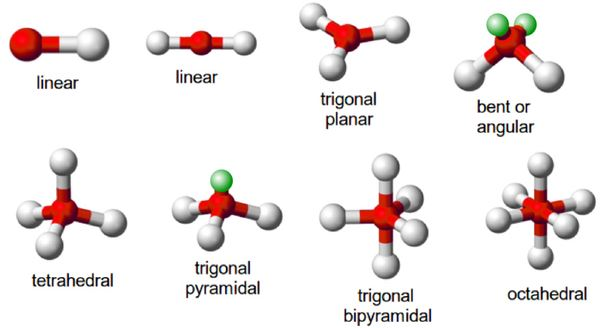# VSEPR Theory

VSEPR theory is the abbreviation of the Valence Shell Electron Pair Repulsion theory and is used to predict the geometry of molecules from the electron pairs that surround the central atoms of the molecule in question.

## What is the VSEPR Theory?

The VSEPR theory is based on the premise that there is a repulsion between the pairs of valence electrons in all atoms, and that the atoms will always tend to arrange themselves in a manner in which this electron pair repulsion is minimalized. This arrangement of the atom determines the geometry of the resulting molecule.

The different geometries that molecules can assume keeping with this theory can be seen in the illustration provided below.The two primary developers of the VSEPR theory are Ronald Nyholm and Ronald Gillespie. This theory is also known as the Gillespie-Nyholm theory to honor these chemists.

In this theory, the repulsion between two electrons caused by the Pauli exclusion principle has greater importance than electrostatic repulsion in the determination of molecular geometry.

### Postulates of VSEPR Theory

The postulates of the valence shell electron pair repulsion theory are listed below.

• In polyatomic molecules (i.e. molecules made up of three or more atoms), one of the constituent atoms is identified as the central atom to which all other atoms belonging to the molecule are linked.
• The total number of valence shell electron pairs decides the shape of the molecule.
• The electron pairs have a tendency to orient themselves in a way that minimizes the electron-electron repulsion between them and maximizes the distance between them.
• The valence shell can be thought of as a sphere wherein the electron pairs are localized on the surface in such a way that the distance between them is maximized.
• Should the central atom of the molecule be surrounded by bond pairs of electrons, then, a symmetrically shaped molecule can be expected.
• Should the central atom be surrounded by both lone pairs and bond pairs of electrons, the molecule would tend to have a distorted shape.
• The VSEPR theory can be applied to each resonance structure of a molecule.
• The strength of the repulsion is strongest in two lone pairs and weakest in two bond pairs.

It can be noted that the strength of the repulsion between a lone pair and a bond pair of electrons lies in between the repulsion between two lone pairs and between two bond pairs.

## Predicting Molecule Shapes with VSEPR Theory

The following steps must be followed in order to decide the shape of a molecule using the VSEPR theory.

• The least electronegative atom must be selected as the central atom (since this atom has the highest ability to share its electrons with the other atoms belonging to the molecule).
• The total number of electrons belonging to the outermost shell of the central atom must be counted.
• The total number of electrons belonging to other atoms and used in bonds with the central atom must be counted.
• These two values must be added in order to obtain the valence shell electron pair number (or the VSEP number)

The VSEP number describes the shape of the molecule, as described in the table provided below.

 VSEP Number The Shape of the Molecule 2 Linear 3 Trigonal Planar 4 Tetrahedral 5 Trigonal Bipyramidal 6 Octahedral 7 Pentagonal Bipyramidal

Each of these corresponding shapes can also be found in the illustration provided earlier. However, the VSEPR theory cannot be used to obtain the exact bond angles between the atoms in a molecule.

## Limitations of the VSEPR Theory

Some important shortcomings of the VSEPR theory include:

• This theory fails to explain isoelectronic species (i.e. elements having the same number of electrons). According to the valence shell electron pair repulsion theory, the shapes of molecules are determined by the number of lone pairs and bond pairs of electrons. However, these species may vary in shapes despite having the same number of electrons.
• The VSEPR theory does not shed any light on the compounds of transition metals. The structure of several such compounds cannot be correctly described by this theory. This is because the VSEPR theory does not take into account the associated sizes of the substituent groups and the lone pairs that are inactive.
• Another limitation of this theory is that it predicts that halides of group 2 elements will have a linear structure, whereas their actual structure is a bent one.

Thus the VSEPR theory can be used to accurately predict the structure of many compounds. To learn more about this theory in a more interactive manner, download the BYJU’S app from the Google Play Store.

#### Practise This Question

Consider the molecules CH4, NH3 and H2O. Which of the given statements is false?